# Appendix

## Occlusion-compatible scanning-order

The following summary presents only a concept which could not be fully verified at the time of writing the thesis.

Consider two 3D scene points $$\boldsymbol{P}_1$$ and $$\boldsymbol{P}_2$$, which are projected onto a target image (virtual view) at the same pixel position $$p$$, and onto the reference view at pixel positions $$\boldsymbol{p}_1'$$ and $$\boldsymbol{p}_2'$$ (see Figure 4.8). To perform an occlusion-compatible scanning order of the reference image, it is necessary to scan first the background point $$\boldsymbol{P}_1$$ and then the foreground $$\boldsymbol{P}_2$$. Hence, when projecting the 3D points $$\boldsymbol{P}_1$$ and $$\boldsymbol{P}_2$$ onto the reference view, it can be derived that $|\boldsymbol{M}'\boldsymbol{P}_2-\boldsymbol{M'C}|<|\boldsymbol{M}'\boldsymbol{P}_1-\boldsymbol{M'C}|, (A.1)$ which is equivalent to the same inequality using pixels in the reference image, thus $|\lambda_2'\boldsymbol{p}_2'-\lambda_e' \boldsymbol{e'}|<|\lambda_1'\boldsymbol{p}_1'-\lambda_e' \boldsymbol{e}'|, (A.2) \label{eq:distance2}$ where $$\boldsymbol{e'}$$ corresponds to the epipole in the reference view. The points $$\boldsymbol{p}_1'$$ and $$\boldsymbol{p}_2'$$ are the projections of the background and foreground 3D points, respectively. Points $$\boldsymbol{p}_1'$$ and $$\boldsymbol{p}_2'$$ are therefore background and foreground pixels. By normalizing/dividing Equation ([eq:distance2]) with the homogeneous scaling factor $$\lambda_e'$$, two cases can be distinguished. First, assuming $$\lambda_e'>0$$, to perform the warping of background pixels $$\boldsymbol{p}_1$$ prior to foreground pixels $$\boldsymbol{p}_2$$, it is sufficient to scan the reference image from the border of the image towards the epipole $$e'$$. Second, in the case $$\lambda_e'<0$$, the reference image should be scanned from the epipole towards the borders of the image.

## “Breakdancers” and “Ballet”

Figure A.1 and Figure A.2 illustrate the multi-view sequences “Breakdancers” and “Ballet”, respectively. These test sequences were acquired by Microsoft Research . Table A.1 and Table A.2 provide the camera calibration parameters. The resolution of both sequences is $$1024 \times 768$$ pixels and the frame rate is 15 frames per second. The calibration parameters were converted into a right-handed coordinate system with the origin of the image located at the top left of the image (see Section 2.2.3).Figure A.1 Camera 3 of the sequence “Breakdancers”.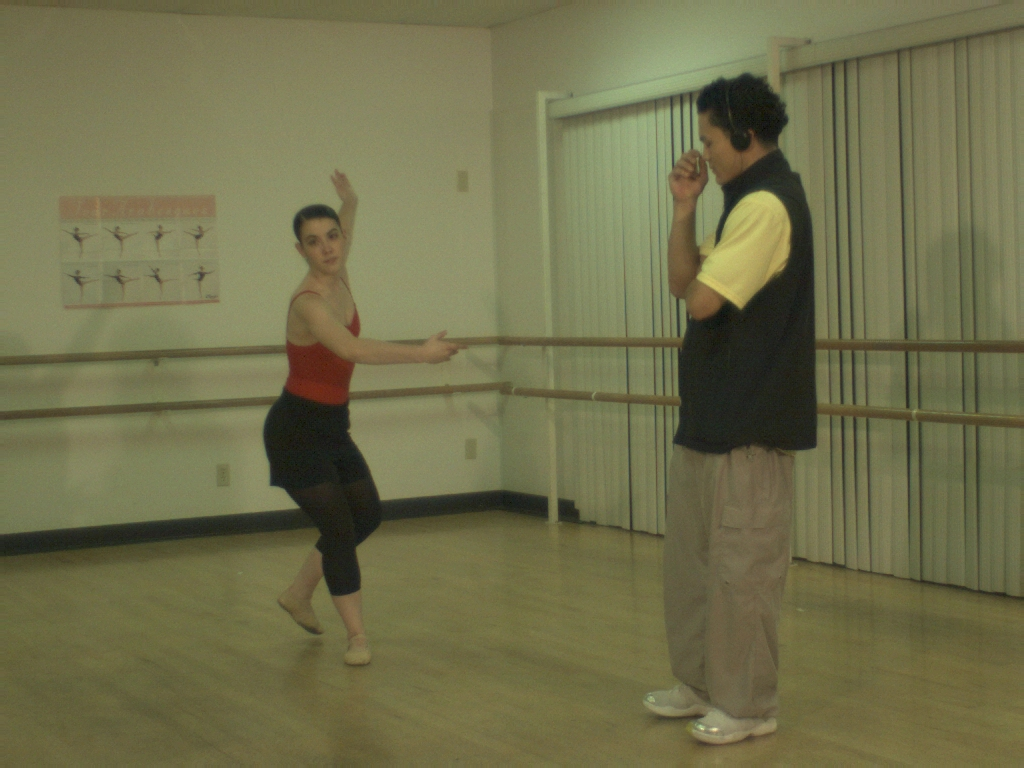Figure A.2 Camera 3 of the sequence “Ballet”.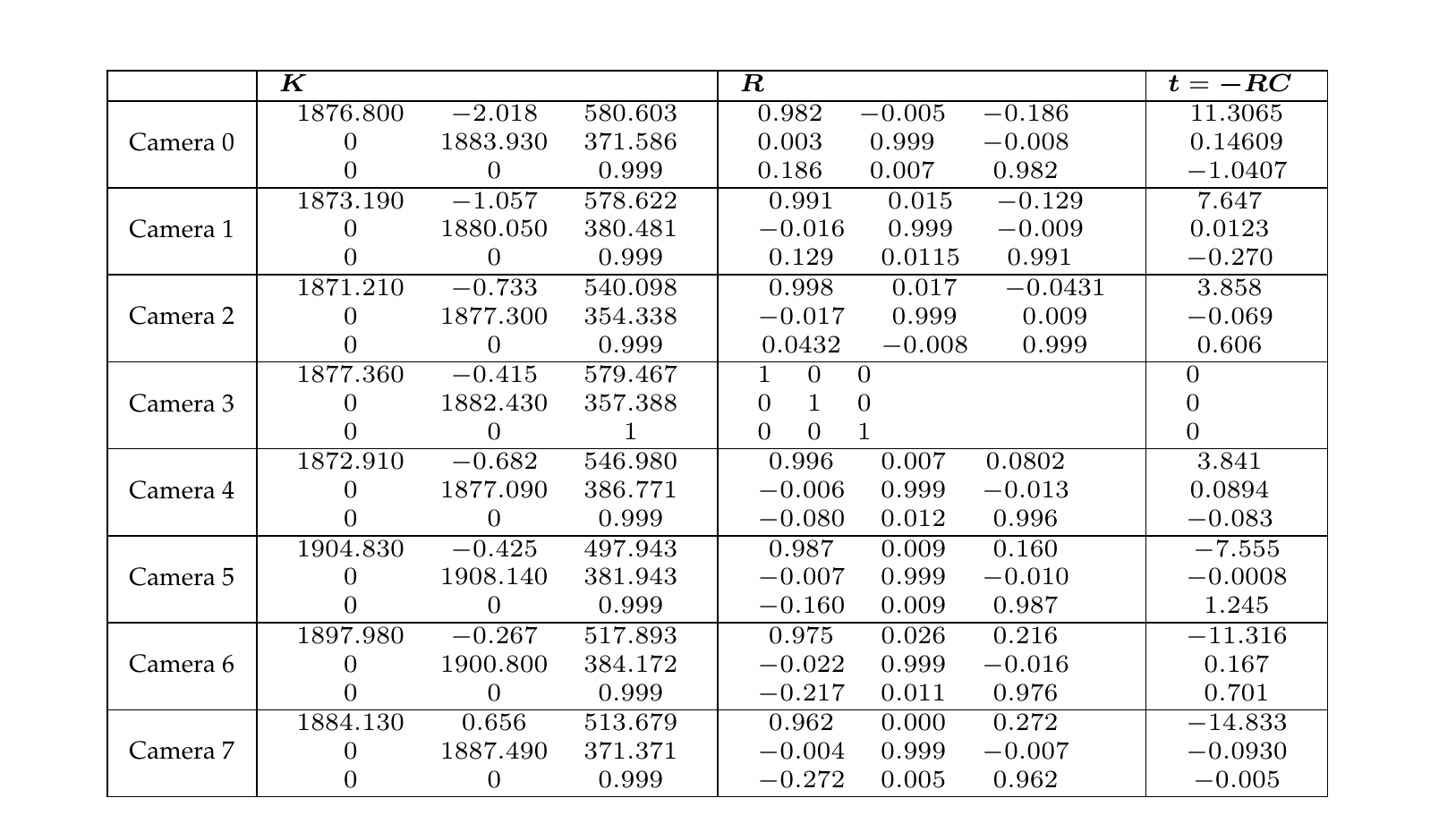Table A.1 Calibration parameters of the multi-view sequence “Breakdancers”.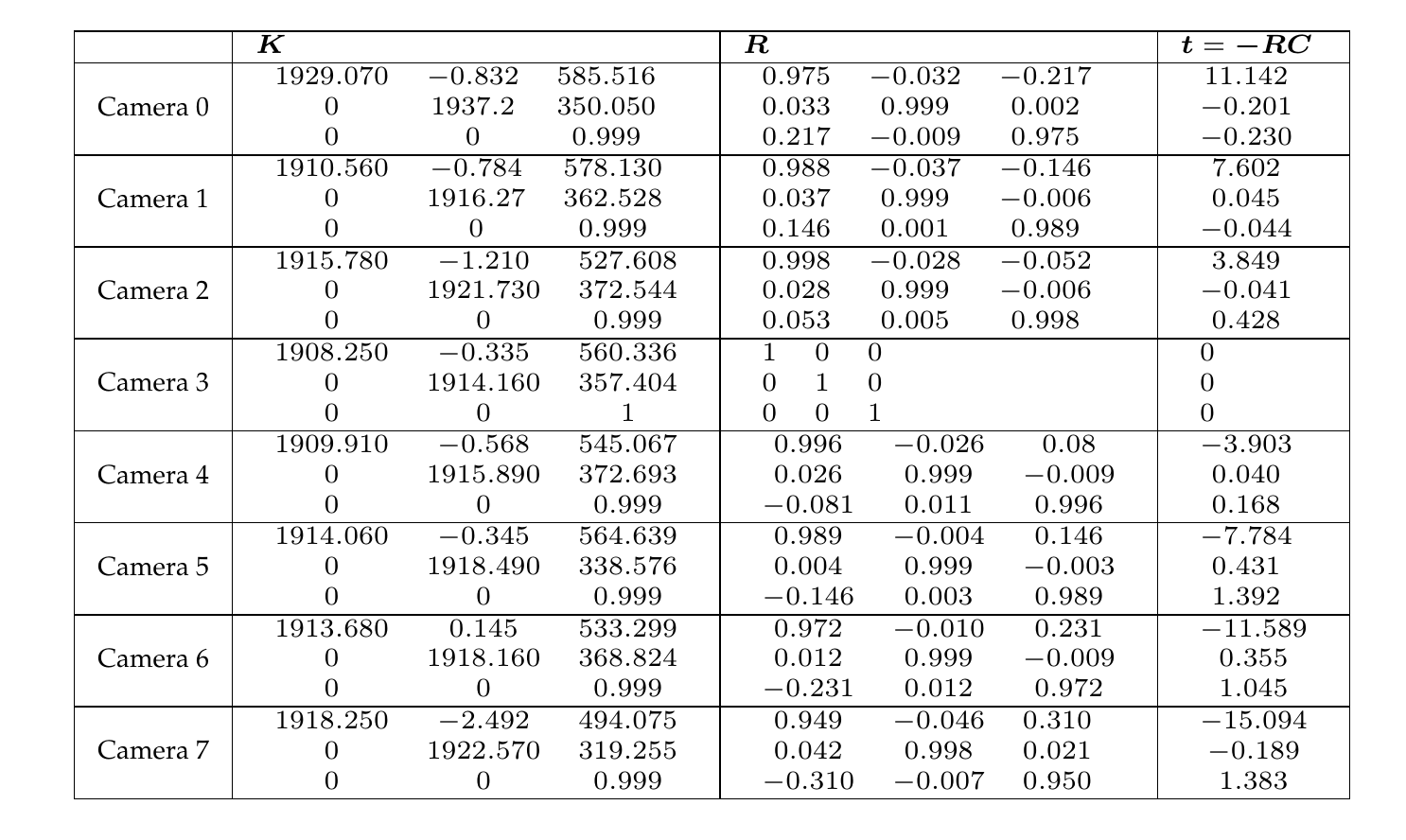Table A.2Calibration parameters of the multi-view sequence “Ballet”.

## “Livingroom”

Figure [figure:Livingroom] illustrates the multi-view images “Livingroom” and Table A.3 provides the camera calibration parameters. The data set was acquired and calibrated by the author. The acquisition of the images was performed using a low cost digital still camera. The radial lens distortion parameters are $$k_1=8.06244e-08$$, $$o_x=512$$ and $$o_y=384$$.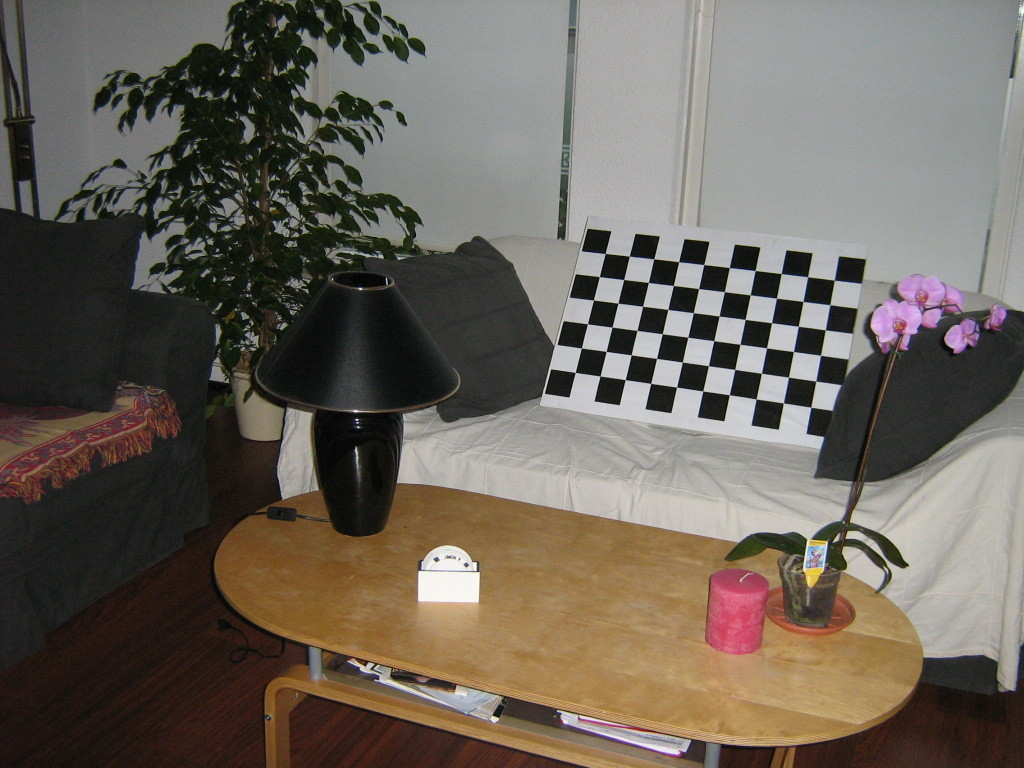Figure A.3 Camera 3 of the multi-view images “Livingroom”.

nTable A.3 Calibration parameters of the multi-view images “Livingroom”.

## “Cones” and “Teddy”

Figure A.4 illustrates the multi-view images “Cones” and “Teddy”, respectively. These multi-view images are available at the Stereo Vision research page  and are provided without calibration parameters. The resolution of the images is $$450 \times 375$$ pixels.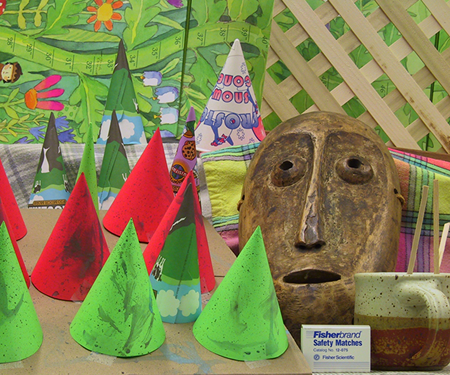(a) (b) Figure A.4 Leftmost view of the multi-view images (a) “Cones” and (b) “Teddy”.

# References

 D. Scharstein, R. Szeliski, and R. Zabih, “A taxonomy and evaluation of dense two-frame stereo correspondence algorithms,” in IEEE workshop on stereo and multi-baseline vision, 2001, pp. 131–140.

 C. L. Zitnick, S. B. Kang, M. Uyttendaele, S. Winder, and R. Szeliski, “Microsoft Research 3D Video Download.” http://research.microsoft.com/en-us/um/people/sbkang/3dvideodownload, last visited: January 2009.